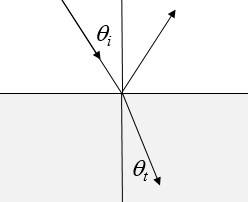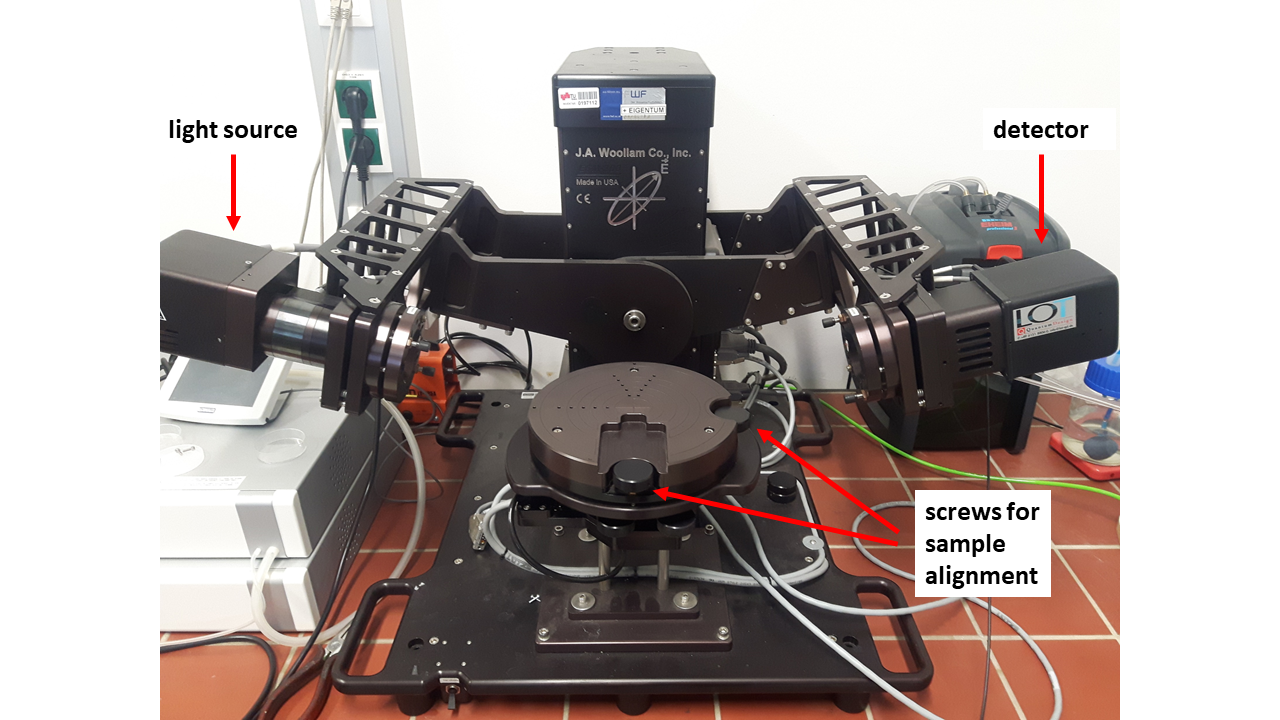Dielectric function
The relative dielectric function describes the relationship between the electric displacement $\vec{D}$ and the electric field $\vec{E}$, $\vec{D}=\epsilon_r \epsilon_0 \vec{E}= \vec{P}+\epsilon_0 \vec{E}$.

 $\epsilon_r\left(\omega \right)$ $\omega$ [1015 rad/s]

There are two conventions for dielectric function. Either it is assumed that the time dependence of $\vec{D}$, $\vec{P}$, and $\vec{E}$ is $\exp (-i\omega t)$ and the plot of the dielectric function looks as it is shown above, or it is assumed that the time dependence of $\vec{D}$, $\vec{P}$, and $\vec{E}$ is $\exp (i\omega t)$ and the imaginary part of the has the opposite sign as in the plot above. Here we will assume a time dependence of $\exp (-i\omega t)$.

Electric susceptibility
The electric susceptibility $\chi_E$ describes the relationship between the polarization $\vec{P}$ and the electric field $\vec{E}$, $\vec{P} = \epsilon_0\chi_E\vec{E}$.

$\begin{equation} \chi_E= \epsilon_r -1 \end{equation}$
 $\chi_E\left(\omega \right)$ $\omega$ [1015 rad/s]

Complex conductivity
Assuming that the electric field has a harmonic dependence, $E=E_0 \exp(-i\omega t)$, then the polarization will also have a harmonic dependence, $P = \epsilon_0\chi_E E_0 \exp(-i\omega t)$. The polarization is the charge density $n$ times the charge $q$ times the displacement $x$, $P = nqx = \epsilon_0\chi_E E_0 \exp(-i\omega t)$. Differentiating this with repect to time yields a relationship between the current density $j$ and the electric field, $j = nq\frac{dx}{dt} = -i\omega \epsilon_0\chi_E E$. Since the current density is related to the electric field by the conductivity, $j=\sigma E$, the conductivity is,

$\begin{equation} \sigma\left(\omega \right)=-i\omega \epsilon_0 \chi_E(\omega). \end{equation}$

 σ(ω)[103 Ω-1 m-1] $\omega$ [1015 rad/s]

The dielectric function, the electric susceptibility, and the complex conductivity are three different ways to present the same information. Usually for frequencies below about 1THz, we use the complex conductivity and for higher frequencies we use the dielectric function or the electric susceptibility.

The index of refraction $n$ and the extinction coefficient $K$
For nonferromagnetic materials where the relative permeability is approximately 1 $(\mu_r\approx 1)$, the real and imaginary parts of the square root of the dielectric function are the index of refraction and the extinction coefficient.

$\begin{equation} \sqrt{\epsilon_r}= n+iK \end{equation}$

When waves travel from vacuum into some material, the frequency remains constant. A plane wave moving to the right in vaccuum has the form $\exp\left(i\left(\omega x/c -\omega t\right)\right)$ where $c$ is the speed of light in vacuum. When this wave enters some material, $c \rightarrow c/ \left(n+iK\right)$. The speed of the electromagnetic waves is smaller than the speed of light in vacuum by a factor of $n$. The extinction coeffcient describes the exponential decay of the amplitude of the electromagnetic waves. For waves propagating in the $x$-direction, the amplitude decays like $\exp\left(-\omega Kx/c \right)$.

 $\sqrt{\epsilon_r}$ $\omega$ [1015 rad/s]

Absorption coefficient $\alpha$
The absorption coefficient describes how the intensity of the light decays. Since the intensity is proportional to the amplitude of the waves squared, the exponential decay of the intensity is $I= I_0\exp\left(-\alpha x\right)$ where,

$\begin{equation} \alpha =\frac{2\omega K}{c} \end{equation}$

 $\alpha$ [106 m-1] $\omega$ [1015 rad/s]

Reflection
The reflection coefficient of light striking the crystal normal to the surface from vacuum ($\epsilon_r=1$) is,

$\begin{equation} R=\frac{\left(n-1\right)^2+K^2}{\left(n+1\right)^2+K^2} \end{equation}$

 $R$ $\omega$ [1015 rad/s]

Reflection at angles other than normal ($\theta_i \ne 0)$
When light strikes an interface between two materials at an incident angle $\theta_i$ from the normal, some of the light is reflected and some is transmitted at an angle $\theta_t$.The the transmitted angle $\theta_t$ is given by Snell's law $\sqrt{\epsilon_i}\sin\theta_i = \sqrt{\epsilon_t}\sin\theta_t$. The amount that is reflected and the amount that is transmitted depends on the polarization of the incident light. If the polarization is parallel to the plane of incidence, it is called p-polarization. If the light is polarized perpendicular to the plane of incidence it is called s-polarization (s for the German word for perpendicular, senkrecht). The incident light ray and the reflected light ray define the plane of incidence. This decomposition into p- and s-polarizations is convenient because these polarizations do not change upon reflection. Incident p-polarized light produces a p-polarized reflection. Incident s-polarized light produces a s-polarized reflection. The polarizations of the electric field can be written as a two-dimensional vector that is perpendicular to the propagation of the electromagnetic wave,

\begin{equation} \vec{E}_i= \begin{pmatrix} |E_{ip}| e^{i \delta_{ip}}\\ |E_{is}| e^{i \delta_{is}}\\ \end{pmatrix}, \qquad \vec{E}_r= \begin{pmatrix} |E_{rp}| e^{i \delta_{rp}}\\ |E_{rs}| e^{i \delta_{rs}} \end{pmatrix}. \end{equation}

Here $E_{ip}$ is the amplitude of the p-polarization of the incident wave, $E_{rp}$ is the amplitude of the p-polarization of the reflected wave, $E_{is}$ is the amplitude of the s-polarization of the incident wave, $E_{rs}$ and the amplitude of the s-polarization of the reflected wave. The reflectance coefficients can be calculated from Maxwell's equations. The results of this calculation are called the Fresnel equations,

$\begin{equation} r_p=\frac{|E_{rp}| e^{i \delta_{rp}}}{|E_{ip}| e^{i \delta_{ip}}}=\frac{\sqrt{\epsilon_t}\cos \theta_i-\sqrt{\epsilon_i}\cos \theta_t}{\sqrt{\epsilon_t}\cos \theta_i+\sqrt{\epsilon_i}\cos \theta_t}, \end{equation}$ $\begin{equation} r_s=\frac{|E_{rs}| e^{i \delta_{rs}}}{|E_{is}| e^{i \delta_{is}}}=\frac{\sqrt{\epsilon_i}\cos \theta_i-\sqrt{\epsilon_t}\cos \theta_t}{\sqrt{\epsilon_i}\cos \theta_i+\sqrt{\epsilon_t}\cos \theta_t}. \end{equation}$

The reflectance coefficients are generally complex meaning that the wave changes its phase upon reflection. In the plots below, it is assumed that the permeabilities are the same in both media $(\mu_i = \mu_t=1)$ and $\epsilon_i = 1$.

$\theta_i=$°

 $r_p$ $r_s$ $\omega$ [1015 rad/s] $\omega$ [1015 rad/s]

Polarization dependent reflection and transmission
The polarization dependent reflection and transmission coefficients are,

$\large R_p=|r_p|^2,$    $\large T_p=1-R_p,$   $\large R_s=|r_s|^2,$    $\large T_s=1-R_s,$    $\theta_i=$45°.

 $R_p,R_s$ $T_p,T_s$ $\omega$ [1015 rad/s] $\omega$ [1015 rad/s]

Ellipsometry
An ellipsometer is an instrument that measures the ratio of the reflectance coefficients when light reflects of a surface. Generally this ratio is a complex quantity and it is typically expressed in terms of two real quantities $\Phi$ and $\Delta$ called the ellipsometry angles.

\begin{equation} \label{eq1} \rho = \frac{r_p}{r_s} = \tan(\Phi) e^{i\Delta} \end{equation}

Here $\tan(\Phi) = \left[(|E_{rp}|/|E_{ip}|)/(|E_{rs}|/|E_{is}|)\right]$ and $\Delta = (\delta_{rp}-\delta_{ip})-(\delta_{rs}-\delta_{is})$ is the phase difference between the p-polarized and s-polarized waves.

Below is a picture of an ellipsometer. The light source and the detector are mounted on two mechanical arms that can move to change the angle $\theta$ of the incident and reflected light.See:
J.A. Woollam - Ellipsometry Tutorial for an explanation of how an ellipsometer works.

Null Ellipsometry
Theoretically you could measure the ellipsometry angles by measuring the amplitude difference and the phase change when light reflects off the surface. However, it is experimentally difficult to measure the phase shift because the frequencies are so high. There is a technique called null ellipsometry where you can measure both ellipsometry angles without a fast measurment of the phase. Unpolarized light is passed through a linear polarizer that sets the amplitude of the p- and s-polarizations. This linearly polarized light then pases through a 1/4 wave plate that consists of a birefringent material that has a fast and a slow axis which are aligned with the p- and s- polarizations. The thickness of the plate is chosen so that it introduces a phase shift of 90° between the p- and s-polarizations. If the polarizer is at 45° from the plane of incidence, the amplitudes of the p- and s- polarizations will be the same and the light will be circularly polarized after the 1/4 wave plate. If the polarizer is set so the amplitudes of the p- and s- polarizations are different, the light is elliptically polarized after the 1/4 wave plate. The elliptically polarized light reflects off the surface and in general produces a reflection that is also elliptically polarized. This light is analyzed by rotating another polarizer in front of the detector. Because the light is elliptically polarized, the intensity measured at the detector has a maximum and a minimun as the analyzing polarizer is turned. The polarizer on the source side is then adjusted and the analyzing polarizer is rotated looking for the condition that the minimum intensity at the detector goes to zero. If the intensity at the detector goes to zero for some angle of the analyzing polarizer, then the reflected light is linearly polarized and the ellipsometry angles can be determmined from the settings of the polarizers.

Ellipsometry equation
Using the relationships $\sqrt{\epsilon_t}\sin(\theta_t)=\sqrt{\epsilon_i}\sin(\theta_i)$ and $\cos(x)=\sqrt{1-\sin^2(x)}$, $\rho$ can be written,

$$\rho = \frac{r_p}{r_s} =\frac{\sin^2\theta_i - \cos\theta_i\sqrt{\epsilon_t/\epsilon_i-\sin^2\theta_i}}{\sin^2\theta_i + \cos\theta_i\sqrt{\epsilon_t/\epsilon_i-\sin^2\theta_i}}.$$

For $\epsilon_i = 1$, this can be solved for the dielectric function of the material that the light is reflected from,

$$\epsilon_t=\sin^2\theta_i\left[ 1+\tan^2\theta_i\left( \frac{1-\rho}{1+\rho}\right)\right].$$

This is called the ellipsometry equation. The ellipsometry angles were calculated from the dielectric function and are plotted below. In an experiment, the dielectric function is calculated from the measured ellipsometry angles.

 $\tan(\Phi)$ $\Delta$ [deg] $\omega$ [1015 rad/s] $\omega$ [1015 rad/s]

Often the sample is not a single material but consists of layers. In that case it is possible to fit the data to a model where the light undergoes multiple reflections from the layers and determine the optical constants of all of the layers as well as the layer thicknesses.

The data plotted above is presented in tabular form in the textbox below. There are 12 text columns separated by tabs. The first 4 columns are just different ways to express the photon energy $E = \hbar\omega = hf = hc/\lambda$.

$\omega$ [rad/s]  $f$ [Hz]  $E$ [eV]  $\lambda$ [m]  $\text{Re}[\epsilon_r]$  $\text{Im}[\epsilon_r]$  $\text{Re}[\chi_E]$  $\text{Im}[\chi_E]$  $n$  $K$  $\alpha$ [m-1]  $R$